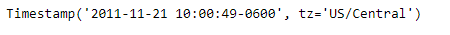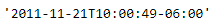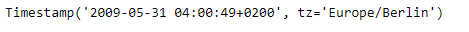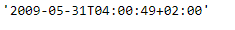# Python | Pandas Timestamp.isoformat

Python is a great language for doing data analysis, primarily because of the fantastic ecosystem of data-centric python packages. Pandas is one of those packages and makes importing and analyzing data much easier.

Pandas` Timestamp.isoformat()` function is used to convert the given Timestamp object into the ISO format.

Syntax :Timestamp.isoformat()

Parameters : None

Return : date time as a string

Example #1: Use `Timestamp.isoformat()` function to convert the date in the given Timestamp object to ISO format.

 `# importing pandas as pd ` `import` `pandas as pd ` ` `  `# Create the Timestamp object ` `ts ``=` `pd.Timestamp(year ``=` `2011``,  month ``=` `11``, day ``=` `21``, ` `                  ``hour ``=` `10``, second ``=` `49``, tz ``=` `'US/Central'``) ` ` `  `# Print the Timestamp object ` `print``(ts) `

Output :Now we will use the `Timestamp.isoformat()` function to convert the date in the given Timestamp object to ISO format.

 `# convert to ISO format ` `ts.isoformat() `

Output :As we can see in the output, the `Timestamp.isoformat()` function has returned the date in the ISO format.

Example #2: Use `Timestamp.isoformat()` function to convert the date in the given Timestamp object to ISO format.

 `# importing pandas as pd ` `import` `pandas as pd ` ` `  `# Create the Timestamp object ` `ts ``=` `pd.Timestamp(year ``=` `2009``, month ``=` `5``, day ``=` `31``, ` `                  ``hour ``=` `4``, second ``=` `49``, tz ``=` `'Europe/Berlin'``) ` ` `  `# Print the Timestamp object ` `print``(ts) `

Output :Now we will use the `Timestamp.isoformat()` function to convert the date in the given Timestamp object to ISO format.

 `# convert to ISO format ` `ts.isoformat() `

Output :As we can see in the output, the `Timestamp.isoformat()` function has returned the date in the ISO format.

Whether you're preparing for your first job interview or aiming to upskill in this ever-evolving tech landscape, GeeksforGeeks Courses are your key to success. We provide top-quality content at affordable prices, all geared towards accelerating your growth in a time-bound manner. Join the millions we've already empowered, and we're here to do the same for you. Don't miss out - check it out now!

Previous
Next